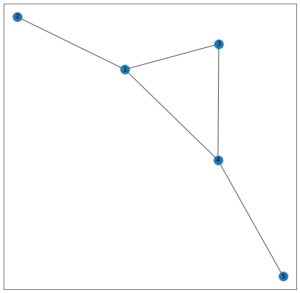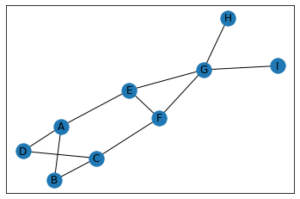Related Articles

# Link Prediction – Predict edges in a network using Networkx

• Last Updated : 08 May, 2020

Link Prediction is used to predict future possible links in a network. Link Prediction is the algorithm based on which Facebook recommends People you May Know, Amazon predicts items you’re likely going to be interested in and Zomato recommends food you’re likely going to order.

 import networkx as nximport matplotlib.pyplot as plt  G = nx.Graph()G.add_edges_from([(1, 2), (1, 3), (1, 4), (3, 4), (4, 5)])  plt.figure(figsize =(10, 10))nx.draw_networkx(G, with_labels = True)

Output:The following means can be assumed in order to successfully predict edges in a network :

2. Jaccard Coefficient
3. Resource Allocation Index
5. Preferential Attachment
6. Community Common Neighbor
7. Community Resource Allocation

If two vertices are connected to the same third vertices, the tendency for them to share a connection is Triadic Closure.

comm_neighb(X, Y) = |N(X)N(Y)|, where N(X) is the set of all neighbours of X.

 import networkx as nx  G = nx.Graph()G.add_edges_from([(1, 2), (1, 3), (1, 4), (3, 4), (4, 5)])e = list(G.edges())  def triadic(e):  new_edges = []    for i in e:    a, b = i      for j in e:      x, y = j        if i != j:        if a == x and (b, y) not in e and (y, b) not in e:          new_edges.append((b, y))        if a == y and (b, x) not in e and (x, b) not in e:          new_edges.append((b, x))        if b == x and (a, y) not in e and (y, a) not in e:          new_edges.append((a, y))        if b == y and (a, x) not in e and (x, a) not in e:          new_edges.append((a, x))    return new_edges  print("The possible new edges according to Triadic closure are :")print(triadic(e))

Output:

The possible new edges according to Triadic closure are :
[(2, 3), (2, 4), (3, 2), (4, 2), (1, 5), (3, 5), (5, 1), (5, 3)]


## Jaccard Coefficient :

It is calculated by number of common neighbors normalized by total number of neighbors. It is used to measure the similarity between two finite sample sets, and is defined as the size of the intersection divided by the size of the union of the sample sets.

Jaccard Coefficient(X, Y) =import networkx as nx  G = nx.Graph()G.add_edges_from([(1, 2), (1, 3), (1, 4), (3, 4), (4, 5)])  print(list(nx.jaccard_coefficient(G))

Output:

[(1, 5, 0.3333333333333333), (2, 3, 0.5), (2, 4, 0.3333333333333333), (2, 5, 0.0), (3, 5, 0.5)]

The jaccard_coefficient built-in function of Networkx necessarily returns a list of 3 tuples (u, v, p), where u, v is the new edge which will be added next with a probability measure of p (p is the Jaccard Coefficient of nodes u and v).

## Resource Allocation Index :

Among a number of similarity-based methods to predict missing links in a complex network, Research Allocation Index performs well with lower time complexity. It is defined as a fraction of a resource that a node can send to another through their common neighbors.

Research Allocation Index(X, Y) =import networkx as nx  G = nx.Graph()G.add_edges_from([(1, 2), (1, 3), (1, 4), (3, 4), (4, 5)])  print(list(nx.resource_allocation_index(G)))

Output:

[(1, 5, 0.3333333333333333), (2, 3, 0.3333333333333333), (2, 4, 0.3333333333333333), (2, 5, 0), (3, 5, 0.3333333333333333)]

The networkx package offers an in-built function of resource_allocation_index which offers a list of 3 tuples (u, v, p), where u, v is the new edge and p is the resource allocation index of the new edge u, v.

This measure was introduced in 2003 to predict missing links in a Network, according to the amount of shared links between two nodes. It is calculated as follows:

Adamic Adar Index(X, Y) =import networkx as nx  G = nx.Graph()G.add_edges_from([(1, 2), (1, 3), (1, 4), (3, 4), (4, 5)])  print(list(nx.adamic_adar_index(G)))

Output:

[(1, 5, 0.9102392266268373), (2, 3, 0.9102392266268373), (2, 4, 0.9102392266268373), (2, 5, 0), (3, 5, 0.9102392266268373)]

The networkx package offers an in-built function of adamic_adar_index which offers a list of 3 tuples (u, v, p) where u, v is the new edge and p is the adamic adar index of the new edge u, v.

## Preferential Attachment :

Preferential attachment means that the more connected a node is, the more likely it is to receive new links (refer to this article for referring to Barabasi Albert graph formed on the concepts of Preferential Attachment) Nodes with higher degree gets more neighbors.

Preferential Attachment(X, Y) = |N(X)|.|N(Y)|
 import networkx as nx  G = nx.Graph()G.add_edges_from([(1, 2), (1, 3), (1, 4), (3, 4), (4, 5)])  print(list(nx.preferential_attachment(G)))

Output:

[(1, 5, 3), (2, 3, 2), (2, 4, 3), (2, 5, 1), (3, 5, 2)]


The networkx package offers an in-built function of preferential_attachment which offers a list of 3 tuples (u, v, p) where u, v is the new edge and p is the preferential attachment score of the new edge u, v.

## Community Common Neighbor :

Number of common neighbors with bonus for neighbors in same community. For applying this, we have to specify the community of all nodes.

Community Common Neighbors(X, Y) = |N(X)N(Y)| +,
where f(u) = 1, if u is in a community; otherwise 0.

 import networkx as nximport matplotlib.pyplot as plt  G = nx.Graph()G.add_node('A', community = 0)G.add_node('B', community = 0)G.add_node('C', community = 0)G.add_node('D', community = 0)G.add_node('E', community = 1)G.add_node('F', community = 1)G.add_node('G', community = 1)G.add_node('H', community = 1)G.add_node('I', community = 1)  G.add_edges_from([('A', 'B'), ('A', 'D'), ('A', 'E'), ('B', 'C'),                  ('C', 'D'), ('C', 'F'), ('E', 'F'), ('E', 'G'),                              ('F', 'G'), ('G', 'H'), ('G', 'I')])  nx.draw_networkx(G)print(list(nx.cn_soundarajan_hopcroft(G)))

Output:[('I', 'A', 0),
('I', 'C', 0),
('I', 'D', 0),
('I', 'E', 2),
('I', 'H', 2),
('I', 'F', 2),
('I', 'B', 0),
('A', 'H', 0),
('A', 'C', 4),
('A', 'G', 1),
('A', 'F', 1),
('C', 'H', 0),
('C', 'G', 1),
('C', 'E', 1),
('D', 'G', 0),
('D', 'E', 1),
('D', 'H', 0),
('D', 'F', 1),
('D', 'B', 4),
('G', 'B', 0),
('E', 'H', 2),
('E', 'B', 1),
('H', 'F', 2),
('H', 'B', 0),
('F', 'B', 1)]


The networkx package offers an in-built function of cn_soundarajan_hopcroft which offers a list of 3 tuples (u, v, p) where u, v is the new edge and p is the score of the new edge u, v.

## Community Resource Allocation :

Computes the resource allocation index of all node pairs using community information.

Community Resource Allocation(X, Y) =import networkx as nx  G = nx.Graph()  G.add_node('A', community = 0)G.add_node('B', community = 0)G.add_node('C', community = 0)G.add_node('D', community = 0)G.add_node('E', community = 1)G.add_node('F', community = 1)G.add_node('G', community = 1)G.add_node('H', community = 1)G.add_node('I', community = 1)  G.add_edges_from([('A', 'B'), ('A', 'D'), ('A', 'E'), ('B', 'C'),                  ('C', 'D'), ('C', 'F'), ('E', 'F'), ('E', 'G'),                              ('F', 'G'), ('G', 'H'), ('G', 'I')])  print(list(nx.ra_index_soundarajan_hopcroft(G)))

Output:

[('I', 'A', 0),
('I', 'C', 0),
('I', 'D', 0),
('I', 'E', 0.25),
('I', 'H', 0.25),
('I', 'F', 0.25),
('I', 'B', 0),
('A', 'H', 0),
('A', 'C', 1.0),
('A', 'G', 0),
('A', 'F', 0),
('C', 'H', 0),
('C', 'G', 0),
('C', 'E', 0),
('D', 'G', 0),
('D', 'E', 0),
('D', 'H', 0),
('D', 'F', 0),
('D', 'B', 0.6666666666666666),
('G', 'B', 0),
('E', 'H', 0.25),
('E', 'B', 0),
('H', 'F', 0.25),
('H', 'B', 0),
('F', 'B', 0)]


The networkx package offers an in-built function of ra_index_soundarajan_hopcroft which offers a list of 3 tuples (u, v, p) where u, v is the new edge and p is the score of the new edge u, v.

Attention geek! Strengthen your foundations with the Python Programming Foundation Course and learn the basics.

To begin with, your interview preparations Enhance your Data Structures concepts with the Python DS Course. And to begin with your Machine Learning Journey, join the Machine Learning – Basic Level Course

My Personal Notes arrow_drop_up